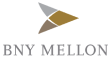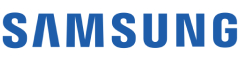New update is available. Click here to update.
Topics

# Kth largest element in the unsorted array

Moderate0/80
Average time to solve is 10m+27 more companies

## Problem statement

You are given an array consisting of 'N' distinct positive integers and a number 'K'. Your task is to find the kth largest element in the array.

Example:
``````Consider the array {2,1,5,6,3,8} and 'K' = 3, the sorted array will be {8, 6, 5, 3, 2, 1}, and the 3rd largest element will be 5.
``````
Note:
``````1) Kth largest element in an array is the kth element of the array when sorted in non-increasing order.

2) All the elements of the array are pairwise distinct.
``````
Detailed explanation ( Input/output format, Notes, Images )
Constraints:
``````1 <= T <= 100
1 <= N <= 10^4
1 <= ARR[i] <= 10^9
1 <= K <= N

Time Limit: 1 sec
``````
Sample Input 1:
``````1
3 1
1 2 3
``````
Sample Output 1:
``````3
``````
Explanation for sample input 1:
``````3 is the first largest element in the array {1,2,3}.
``````
Sample Input 2:
``````1
4 2
5 6 7 8
``````
Sample Output 2:
``````7
``````
Explanation for sample input 2:
``````7 is the second largest element in the array {5,6,7,8}.
``````Console